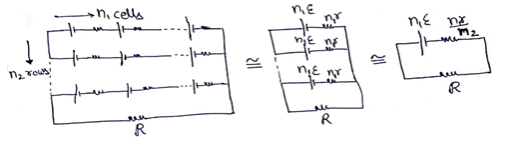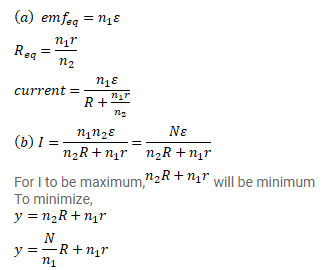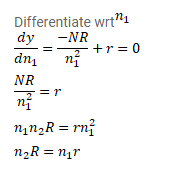Deepak Scored 45->99%ile with Bounce Back Crack Course. You can do it too!

# Consider N = n1n2 identical cells, each of emf ε and internal resistance r.

Question:

Consider $N=n_{1} n_{2}$ identical cells, each of emf $\varepsilon$ and internal resistance $r$. Suppose $\mathrm{n}_{1}$ cells are joined in series to form a line and $\mathrm{n}_{2}$ such lines are connected in parallel. The combination drives a current in an external resistance $R$.

(a) Find the current in external resistance.

(b) Assuming that $\mathrm{n}_{1}$ and $\mathrm{n}_{2}$ can be continuously varied, find the relation between $\mathrm{n}_{1}, \mathrm{n}_{2}, R$ and $r$ for which the current in $\mathrm{R}$ is maximum.

Solution: